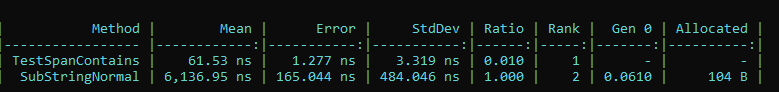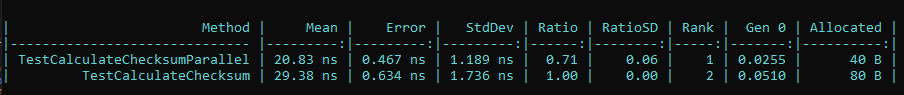# High Performance C#

### 1. Span

Span is a simple data structure to reference some data, it reminds me Rust’s slice which holds a reference and length. Span also has indexer, but the idea is same.

``````public readonly ref struct Span<T>
{
...
}
``````

Let’s say you’re making some operations on some strings, such as splitting or getting substring. Since string in C# is immutable, you’ll be allocating a lot of unnecessary strings. Maybe use a simple for loop, locate the index and length, then create span for your operation.

This code simply finds the substring.

``````public ReadOnlySpan<char> GetSubstring(string strSource, string searchWord)
{
if (!strSource.Contains(searchWord)) return string.Empty;
int End = strSource.IndexOf(searchWord, 0) + searchWord.Length;
return strSource.Substring(0, End - 1).AsSpan();
}

public ReadOnlySpan<char> GetSubStringSpan(string strSource, string searchWord)
{
for(var i = 0; i<span.Length; i++)
{
if (span[i] == searchWord)
{
var k = 0;
for(var y = i; y < searchWord.Length; y++)
{
if (span[y] != searchWord[k])
goto OuterLoop;
k++;
}
return span.Slice(i, searchWord.Length);
}

OuterLoop:
continue;
}
return null;
}
``````There is also Memory type, it’s just like Span except it’s a regular struct instead of ref struct. It means it might get boxed and live on the managed heap. In multi-threaded situations, such-as your method is async, you’ll have to use Memory instead of Span.

### 2. stackalloc

With stackalloc you can create arrays on the stack, but be careful unless you wanna receive an stackoverflowexception. Considering stack size is limited, you shouldn’t create big arrays with that. I usually don’t know how much capacity I need, so it’s rare to use this one.

``````
public void DoSomeArrayOperation()
{
Span<int> arr = stackalloc;
foreach(var val in arr)
{
//some operation
}
}
``````

### 3. SkipLocalsInit

As you know, the variables inside the stack gets zeroed, that’s why local variables becomes default. However, that has a cost. You can use SkipLocalsInit attribute for your method, class or even module. It’s smart, so only non-reference types will not be zeroed. On the other hand, because of no zeroing the variables inside of it might be garbage, don’t forget overriding. It’s handy with stackalloc.

``````
[SkipLocalsInit]
public void DoSomeArrayOperation()
{
Span<int> arr = stackalloc;
foreach(var val in arr)
{
//some operation
}
}
``````

### 4. Pooling

Instead of always allocating a new array, you can reuse by suing ArrayPool class. Bad side of it, you need to return the array explicitly. In some cases you can consider IDisposable.

``````public int SomeOperation()
{
var arrayPool = ArrayPool<int>.Shared;
var buffer = arrayPool.Rent(count);
// doing things
arrayPool.Return(buffer);

return result;
}
``````

You can also consider pooling for objects, but it’s only suggested when you create some heavy objects a lot.

### 5. AllocateUninitializedArray

You can use AllocateUninitializedArray to avoid zeroing while allocating a new big array, if it’s small, it will create a regular array. The values inside of it might be garbage, but you can override.

``````var data = GC.AllocateUninitializedArray<int>(1024);
``````

### 6. Data Locality

To utilize caching and considering memory access patterns, sequential access is very much helpful for performance. For instance sometimes you can use list of struct type instead of class. Also to add a few more things about list, 1. the capacity of the list grows like 2-4-8-16-32… with every value inside of it gets copied into a new list. So try to have an enough initial capacity as much as possible. 2. Prefer array over list, direct access is faster than using vtable.

### 7. Loop Unrolling

This is a trick I got from the book Street Coder. An instruction in CPU is processed in a few units, in this video they explain it very clearly, and show that how CPU parallelize the work. It seems like if the computation depends on the previous value, CPU will not be able to parallelize the work as expected.

``````        public int CalculateSomeNumber(int[] array)
{
var result = 0;
for (var i = 0; i < array.Length; i++)
{
result += (array[i] + 1) * 2; // to calculate the next one, you need to finish this first
}
return result;
}
``````

If we increase the gap between depended code, the next instruction does not get blocked for the previous one.

``````        public int CalculateSomeNumberParallel(int[] array)
{
int n0 = 0, n1 = 0, n2 = 0, n3 = 0, result = 0;
int i = 0;
for (; i < array.Length - 4; i += 4)
{
n0 += (array[i + 0] + 1) * 2;
n1 += (array[i + 1] + 1) * 2;
n2 += (array[i + 2] + 1) * 2;
n3 += (array[i + 3] + 1) * 2;
}
for(; i < array.Length; i++)
{
result += (array[i] + 1) * 2;
}
return result + n0 + n1 + n2 + n3;
}
``````

The result is### 8. Returning Empty Array Or List

This one is from Nick Chapsas’s Youtube videos. When you need to return an empty array or list, instead of allocating, you can just return

``````    return Array.Empty<int>();
// or for lists
return Enumerable.Empty<int>();
``````